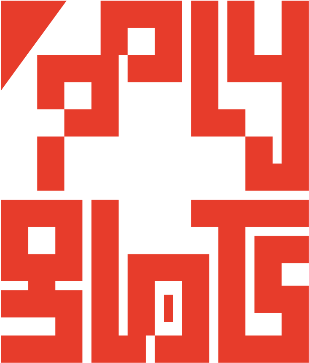polygl0ts

EPFL's CTF teamSquare CTF 2018 - C1: dot-n-dash

Description

The instructions to disable C1 were considered restricted. As a result, they were stored only in encoded form.

The code to decode the instructions was regrettably lost due to cosmic radiation. However, the encoder survived.

Can you still decode the instructions to disable C1?

Analysis

The file instructions.txt contains a series of dots and dashes. In dot-n-dash.html we have the algorithm used to encode it, but not the decoding one.

The encoding algorithm is divided in three steps.

Step 1

var a=[];
for (var i=0; i<input.length; i++) {
var t = input.charCodeAt(i);
for (var j=0; j<8; j++) {
if ((t >> j) & 1) {
a.push(1 + j + (input.length - 1 - i) * 8);
}
}
}

The first for loop iterates over all input characters. The second one on the bits of the current character. For each bit, if its value is 1, 1 + j + (input.length - 1 - i) * 8 (where i is the character index and j the bit index) is added to the output.

In other words, this step is taking the bit representation of the input string and building a list with the indexes of all bits with value 1.

Step 2

var b = [];
while (a.length) {
var t = (Math.random() * a.length)|0;
b.push(a[t]);
a = a.slice(0, t).concat(a.slice(t+1));
}

The code above just randomizes the list obtained after step 1.

Step 3

var r = '';
while (b.length) {
var t = b.pop();
r = r + "-".repeat(t) + ".";
}

This is the final step. It builds a string by going over the elements of the list obtained with the previous two steps, and adding as many - as the value of the current element followed by a ..

So, for example, [1, 3, 4] will give ‘-.—.—-.’.

Solution

We reverse the three steps one by one going backwards.

Step 3

• Split the encoded string on ..
• Count the number - building a list of numbers.

Step 2

• Sort the list.

Step 1

• Build a binary string of all 0s with the correct length.
• For each number in the list, change to 1 the value of the bit at that index.
• Convert the binary string to ASCII.

And we are done!

Instructions to disable C1:
1. Open the control panel in building INM035.
2. Hit the off switch.

Congrats, you solved C1! The flag is flag-bd38908e375c643d03c6.

Code

You can find the script we used to get the flag here.